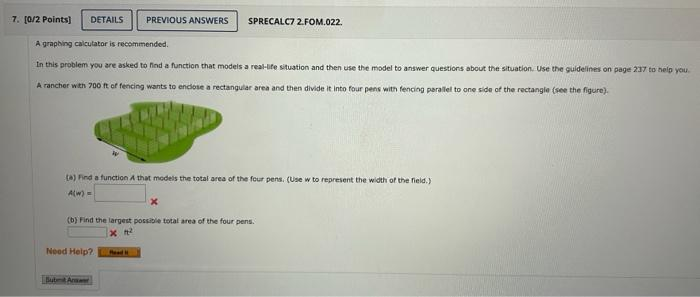# (Solved): Please get the equation right this is last attempt I will leave a thumbs up!! In this problem you ar ...

Please get the equation right this is last attempt I will leave a thumbs up!!In this problem you are asked to find a function that models a real-ife situation and then use the model to answer questions about the situation. Use the guidelines on page 237 to nelp you. A rancher with of fencing wants to enclose a rectangular area and then divide it into four pens with fenciog parallel to one side of the rectangle (see the figure). to) Find a function thut modes the total ares of the four pens. (Use w to represent the width of the field.) (b) Find the largect possigle total area of the four pens.

We have an Answer from Expert